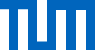What's the cheapest way from left to right?

# The classic among shortest path algorithms

You want to know, how to get from Munich to Cologne as fast as possible? Is the fastest route via Stuttgart or via Frankfurt? Dijkstra's Algorithm can help you! With this algorithm, you can find the shortest path in a graph. The vertices of the graph can, for instance, be the cities and the edges can carry the distances between them.

Dijkstra's Algorithm can also compute the shortest distances between one city and all other cities. And the edges can describe costs, distances, or some other measure that is helpful for the user.

An important restriction of Dijkstra's Algorithm is, that all edge costs (which is how we call the edge labels) must not be negative.

## Legend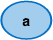Node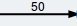Edge with weight 50

## Which graph do you want to execute the algorithm on?

### Modify it to your desire:

• To create a node, make a double-click in the drawing area.
• To create an edge, first click on the output node and then click on the destination node.
• The edge weight is changed with a double-click on the edge.
• Right-clicking deletes edges and nodes.

A occured when reading from file: the contents:

## Legende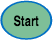Starting node from where distances and shortest paths are computed.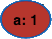Node being processed.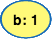Node in the priority queue.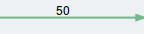"Predecessor edge" that is used by the shortest path to the node.

## Algorithm status

BEGIN

d(v) ← 0

FOR i = 2,..,n DO

d(v[i]) ← ∞, parent(v[i]) ← NULL

WHILE queue ≠ ∅ DO

u = queue.extractMin()

FOR ALL (u,w) ∈ E DO

dist ← d(u) + l(u,w)

IF w ∈ queue AND d(w) > dist DO

d(w) = dist, parent(w) = (u,w)

ELSE IF parent(w) == NULL THEN

d(w) = dist, parent(w) = (u,w)

queue.insert(w,dist)

END

### Priority Queue:

Your browser does not support HTML5 Canvas. Please use a modern browser, i.e.Mozilla Firefox

## Task is terminated if the tab is changed.

You can open another browser window for reading the description in parallel.

## LegendStarting node from where distances and shortest paths are computed.Node that has been chosen correctly.

## Changing the tab aborts the exercise!

You can open another browser window, to read the description in parallel.

## LegendStarting node from where distances and shortest paths are computed.Edge with currect edge costs.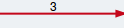Edge with wrong edge costs.

## What are the edge costs?

### Node distances:

Start b c d e f g h i
Initiali-
sation
0
Step 1 3 2 4 34
Step 2 14
Step 3 12 13
Step 4 6 8
Step 5 12 7
Step 6 8 37
Step 7 36
Step 8 35
Step 9 35

## What are the edge costs?

This table shows all distances from the node to the starting node during every round of the algorithm. Some cells in the table are empty.

Can you find out the costs for these edges? Just doubleclick on an edge and set its cost.

## The task is terminated if the tab is changed

You can open another browser window for reading the description in parallel.

## LegendStarting node from where distances and shortest paths are computed.Node being processedNode in the priority queue"Predecessor edge" that is used by the shortest path to the node.

## Test your knowledge: How does the algorithm decide?

BEGIN

d(v) ← 0

FOR i = 2,..,n DO

d(v[i]) ← ∞, parent(v[i]) ← NULL

WHILE queue ≠ ∅ DO

u = queue.extractMin()

FOR ALL (u,w) ∈ E DO

dist ← d(u) + l(u,w)

IF w ∈ queue AND d(w) > dist DO

d(w) = dist, parent(w) = (u,w)

ELSE IF parent(w) == NULL THEN

d(w) = dist, parent(w) = (u,w)

queue.insert(w,dist)

END

## Task is terminated if the tab is changed.

You can open another browser window for reading the description in parallel.